# Bihar Board 12th Physics Important Questions Long Answer Type Part 1

BSEB Bihar Board 12th Physics Important Questions Long Answer Type Part 1 are the best resource for students which helps in revision.

## Bihar Board 12th Physics Important Questions Long Answer Type Part 1

Question 1.
(i) State and prove Gauss’s theorem, (ii) Deduce an expression for the intensity of electric field at a point outside a uniformly charged sphere.
(i) Gauss’s theorem: The total flux of the normal component of electric intensity over a closed surface is equal to $$\frac{1}{\epsilon_{0}}$$ times the total charge ∈0
inside the surface, where eg is the permittivity of the free space.

Proof: Let us consider a point O inside a closed space s and that a charge +q is kept at O. Let r be the distance of a point P of a small are ds from the point O. Hence at P due to charge +q, the intensity of the electric field E will be
E = $$\frac{1}{4 \pi \epsilon_{0}} \cdot \frac{q}{r^{2}}$$ ………….(1)
If the outward draw normal on the area ds from the point P be PN and that direction of the intensity $$\vec{E}$$ makes an angle θ with OP, then the component of $$\vec{E}$$ in the normal direction will be E cosθ.
∴ Intensity in normal direction.
= $$\frac{1}{4 \pi \epsilon_{0}} \frac{q}{r^{2}} \cos \theta$$ [putting the value of E]
Hence the electric flux on the area ds will be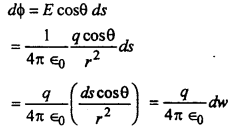where dw is the solid anlge subtended by ds at O and equal to [/latex]\frac{d s \cos \theta}{r^{2}}[/latex]
Hence the total flux passing through the whole surface will be(ii) Intensity of the electric Held at a point due to a charge sphere-
When the point is outside the sphere: Let A be the charged sphere of radius R and Let it be given a charge of +q on the outer surface of the sphere. The charge will be uniformly distributed over it surface. The lines of force will be directed outwards radially.Let P be the point distant r from the centre O of the sphere A where the intensity of the electric field due to the sphere A is to the calculated. Now a sphere B of radius r and centre at O (concentric with the sphere A) is drawn and this is called the Gaussian surface. The lines of force are radially outwards, so the the lines will be every where normal to the Gaussian surface. Hence the electric intensity E is normal at the point P on this surface. Since the area of the sphere B is 4πr2, hence the total electric fulx on its surface = E 4πr2.

But from Gauss’s theorem, the electric flux on the surface of the sphere B,
Φ = $$\frac{1}{\epsilon_{0}}$$ × total internal charge
= $$\frac{1}{\epsilon_{0}}$$ × q
where ε0 is permittivity of the free space.
Hence E × 4πr2 = $$\frac{1}{\epsilon_{0}}$$ × q
or, E = $$\frac{q}{4 \pi \epsilon_{0} r^{2}}$$
This is the required expression at the point P (out side the sphere A).

Question 2.
Draw a levelled diagram of a Van de Graaff generator. Give its principle, construction and working.
Van de Graaff Generator: It is a device used for building up high potential difference of the order of a few million volts. Such a high pot diffs are used to accelerate charged particles like electrons, protons or ions.

Principle: It is based on

• Action of sharp points
• The property that charge given to a hollow conductor is transferred to outer surface and is distributed uniformly over it.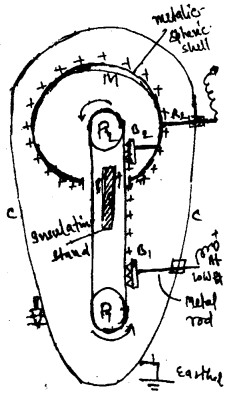Construction: The essential parts of Van de Graaff generator is shown in the fig. It consists of a large metalic spherical shell of radius equal to a few meters. This is spupported on non-conducting stand S at a suitable eight. A long narrow belt of insulating material is wound arround two pullys P1 & P2. P1 is at ground level and P2 is at the centre of M. The belt is kept moving continuously over the pulleys with the help of motor. B1 and B2 are two sharply pointed metal combs fixed as shown B1 is called spray comb and B2 called collecting comb. The whole system is enclosed inside a metalic chamber cc, spherical shell M is connected to a metalic rod R. On which high voltage is obtained.

Working: The spray comb is given a +ve potential (≃ 104 volt) w.r. to the ground by high tension source. Due to discharging action of sharp points a +ve charged electric wind is set up which sprays +ve charge on the belt. As the belt moves and reaches the sphere, a -ve charge is induced on the sharp ends of the collecting comb B2 and equal +ve charge is induced on the farther end of B2. This +ve charge shifts immediately to the outer surface of M. Due to discharging action of sharp point of B2; a – very charged electric wind is set up. This neutralises the +ve charge on the belt. This process is continued and +ve charge on sphere M goes on accumulating.

As the capacity of the sperical shell C = 4π∈0R and V = $$\frac{Q}{C}=\frac{Q}{4 \pi \epsilon_{o} R}$$, R = radius of sphere is cas H.
∴ V ∝ Q. Thus potential of M increases continuously.

The break down field of air is about 3 × 106 V/m. At the moment, the pot of sphere exceeds this value, air around M is ionised and leakage of charge starts the leakage is minimised by housing the generator assembly inside a steel chamber filled with N2 or median at high pressure.

Question 3.
Obtain an expression for the loss of energy when to charged conductors are joined together.
Or, Show that there is loss of energy in shearing of charges.
Loss of energy: Let us consider two conductors A and B of capacities c1 and c2. Let the charge on them be Q1 and Q2 respectively and potentials ν1 and ν2 (supposed ν1 > ν2). When they are connected by a metallic wire let the charged now on the two conductors A and B be respectively Q1‘ and Q2‘ and the common potential be ν.

Now before the two conductors are connected,
ν1 = $$\frac{Q_{1}}{c_{1}}$$ and ν2 = $$\frac{Q_{2}}{c_{2}}$$
If Q be the total charge on both the conductors, then we have
Q = Q1 + Q2 = ν1c1 + ν2c2 …………(1)
Also Q = Q1’ + Q2’ = νc1 + νc2 …………(2)
So from equation (1) and (2) we have
ν(c1 + c2) = ν1c1 + ν2c2
or, ν = $$\frac{v_{1} c_{1}+v_{2} c_{2}}{c_{1}+c_{2}}$$ ………….(3)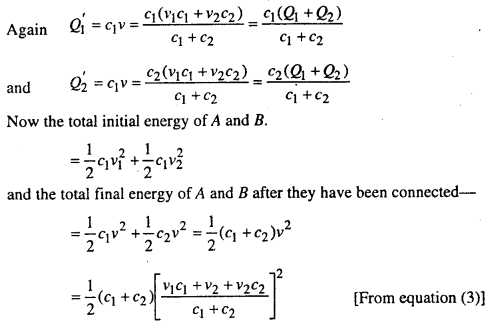Hence the loss of energy due to sharing of charges between the two conductors.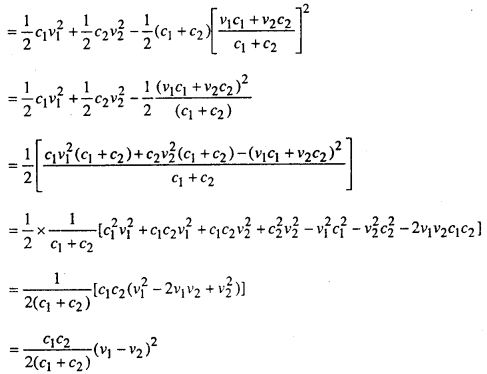Since (ν1 – ν2)2 is always positive, even if ν1 < ν2, so there will always a loss of energy due to sharing of charges between two conductors. This loss of energy appears as heat in the connecting wire and in light and sound when a spark passes.

Question 4.
What is capacitor? Find expression for capacity of a parallel plate capacitor with compound dielectric.
Capacitor or Condenser: The arrangement of conductors in which the capacity of a conductor is artificially increased without increasing its size.

Capacity of a capacitor with compound dielectric: When more than one dielectric medium is inserted between two plates of the capacitor it is called capacitor with compound dielectric or impound capacitor. Let a dielectric slab of thickness t is inserted in the space between two plates A & B of a parallel plate capacitor separated by distance ‘d’.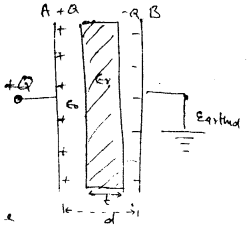It +Q charge is given to its collecting plate A, then its surface density σ = $$+\frac{Q}{A}$$
Thus by coulom’s law, the electric intensity in between the space of two plates in air = E $$\frac{\sigma}{\epsilon_{0}}$$
and that of in the dielectric E2 = $$\frac{\sigma}{\epsilon_{0} \epsilon_{r}}$$
∴ potential diff. between the two paltes A & B of capacitor
V = work done in bring (+1) charge from B to A.
= $$\frac{w}{q}$$
where W = work done (w1) in air + work down (w2) in dielectric = F(d – t) + F’ (t)Note: Capacity is increased on inserting dielectric slab in between the space (air) of the capacitor.

Question 5.
Describe a stretched wire potentiometer. How it is used for comparing the e.m.f. of two cells?
Stretched wire potentiometers: It consists of a uniform wire of length usually 10 metres stretched on a wooden board. Wires bent at each metre length as shown in fig. At the ends of this wire terminals for connection are attached. To measure the length of the wire a parallel scale is attached. A Jockey J is also attached which moves parallel to the scale and it can be made to contact any point on the wire. There is an arrow mark in the Jockey giving the ending of the point of contact on the wire.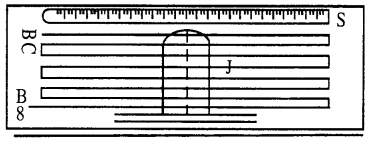Comparison of e-m,f. of two cells: For comparison of the e.m.f. of two cells, the circuit diagram is shown in the figure. AB is a stretched wire potentiometer. A storage cells E, a key K and a rheostat Rh are attached in series and joined to the end A and B of wire. The galvanometer G is connected in series with a resistance R and then connected to a two way key K1 . M and N are two cells whose e.m.f. e1 and e2 are to compared.First of all M cells is brought into circuit. Jockey is moved along the wire till balance is obtained, i.e. deflection in galvanometer will be zero. The length of the wire l1 between A and this point is measured.

Let the current flowing through wire = I
The resistance of wire l1 = l1 · ρ.
where ρ is the resistance per unit length.
e.m.f. of the cell M = current × resistance
i.e. e1 = Il1ρ ……….(1)

Now N cell is brought into circuit. Again no deflection in the galvanometer is obtained by pressing the Jockey on the wire. In this case also the length of the wire l2 between A and this point is measured.

Resistance of wire l2 = l2ρ
Hence e.m.f. of the cell N
= Il2ρ
i.e. e2 = Il2ρ ……….(2)
Eq.(1) + eq.(2)
$$\frac{e_{1}}{e_{2}}=\frac{I l_{1} \rho}{I_{2} \rho}$$
= $$\frac{l_{1}}{l_{2}}$$ ………….(3)
Putting the value of l1 and l2, e.m.f. of cells can be compared.

Question 6.
What is a cyclotron? Discuss its construction, working, theory and limitation.
Cyclotron is a device used to accelerated heavy charged particles like protons and neutrons. This results in the production of high energy beam. Which is then used for artificial disintegration.

Construction: It consists of two D shaped metal boxes called the Dees enclosed in a evaluated chamber. There is a very small gep between these dee’s face across with a high potential difference is applied by a high frequency oscillator. Thus an alternating electric field is established between dees. A source of particles is placed between the dees. The whole apparatus in placed between the pole pieces NS of a strong magnet.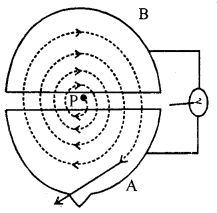Working: Suppose protons p is placed at the centre in the gap between the dees. At this time imagine A to be negative and B to be negative proton will be accelerated by the electric field between the dees towards A. But as it enters inside A. A magnetic field B is acting on it. It will give the ion a circular path. After it takes a certain time t to cover the semi circle, it emerges out of A. I directly at the same time A becomes positive and B becomes negative due to high-frequency oscillator. Thus the proton receives a fresh acceleration in the regain and B with increased velocity. The magnetic field again turns it path into a semicircle of increased radius. This process is repeated again and again. Due to this proton receives high velocity. Its energy will be very high.

Theory: Let us consider a proton of mass m and charge q. The magnetic field $$\vec{B}$$ acts on the dees.
∴ Force on proton = q$$\vec{V} \vec{B}$$
where V is the velocity Consequently, particle moves along a circular path
∴ centripetal force = $$\frac{m v^{2}}{r}$$ ………(2)
where r is the radius of the path
Comparing e.q. (1) and eq. (2) we get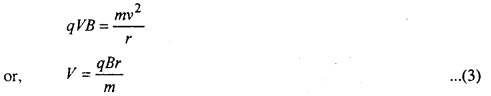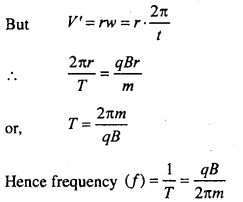This frequency is called cyclotron frequency. It is also called a resonance condition.
If r = R (radius of dees) thenLimitation of Cyclotron:
Here we assumed that even at high velocities mass will be constant. But according to Einstein mass varies with velocity. Hence the mass has increased with the velocity. As velocity increase, it takes a longer time to cover the semi-circular path. This put a limit to the working at the cyclotron to a limited velocity only.

Question 7.
Describe moving coil galvanometer and its working with theory. Write on which factors its sensitivity depends upon.
Moving coil galvanometer: This is simple and sensitive instrument which is used for detecting current in a circuit.

This works on the principle of Fleming’s left hand rule. This is invented by D-Arsonoval. Hence the galv. is also called D-Arsonoval galv.

Construction: It consists of a rectangular coil C over which several turns of insulated fine wire is wound. It is suspended by a thin wire A of phosphor bronze. In the phosphor bronze wire, a small plane or concave mirror M is fitted. Deflection of the coil is measured by lamp and scale arrangement with the help of mirror. The other end of the coil is connected with a spring S of phosphor bronze.

The spring is connected to terminal E. The coil is suspended between poles pieces NS of a strong magnet horse shoe type.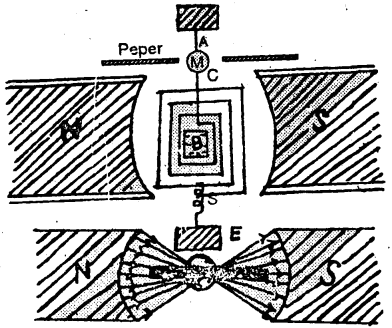When the two terminals D and E are joined in an electrical circuit, the current passes through the coil. Due to this the coil is deflected and phosphor bronze wire is twisted.

The pole pieces of the magnet are made of soft iron and are cylindrically concave in shape. This makes the field radial. A soft iron cylinder B, known as core is placed within coil and is detached from it. The field in the gap is always in an uniform.

Theory: Let us consider a single turn CDEF of the rectangular coil. Let l be the length CD or EF and b the breadth CF or DE. B is magnetic field in which the coil is suspended. Let I be the current passes through the coil.
Force on CD or EF = BIl

The forces upon two opposite CD or EF are equal and opposite. Hence they constitute a couple.
∴ Moment of the couple = BIl . b
If the coil contains n turns, the total moment of the couple
= BIlb . n …………(1)
Due to this couple, the coil is deflected and the suspension wire is twisted. This twist acts as controlling couple.
Let the coil is deflected through an angle θ.
∴ Restoring couple = Kθ ………….(2)
Where K is a constant of the suspension wire.
In equilibritftn state, Eq. (1) = Eq. (2) .
or, Bllb . n = KθThus the current is directly proportional to deflection.
The deflection (θ) is known by mirror M and lamp and scale arrangement.

Sensitivity: From, Eq. (3)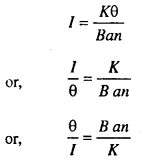Here $$\frac{\theta}{I}$$ is called the sensitivity of the Galvanometer.

Hence for high sensitivity- (i) B should be large. For this purpose a powerful magnet is used.

• a i.e. area of the coil should be large.
• n i.e. number of turns in the coil should be greater.
• Kshould be low. Hence to reduce K, the phosphor bronze is made flat.

Question 8.
What do you understand by electro magnetic induction?
State and explain Faraday’s law of electro magnetic induction.
Electro magnetic induction: On the basis of experiments, Faraday concluded that there is a close relationship between electricity and magnetism. Faraday showed that a magnet is moved towards or away from a coil an e.m.f. is produced. Hence a current flows through the coil. This current lasts so long as there is relative motion between the magnet and coil. This current is called “Induced current” and the e.m.f. is called “Induced e.m.f.” Thus phenomenon is known as “Electromagnetic induction.”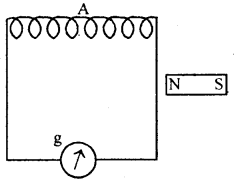A is a coil near which there lies a magnet NS. The coil is connected to a galvanometer G when the magnet is suddenly moved towards the coil a deflection in the galvanometer is observed. Hence a current flows through the coil. If the magnet is taken away from the coil, the galvanometer shows a deflection in opposite direction.

Faraday’s Law of Electron Magnetic Induction:
First law: Whenever in a closed circuit the magnetic lines of force passing through the circuit change an e.m.f. is induced in the circuit and it will remain in the circuit so long as the changes taken place.

Second law: The induced e.m.f. in a coil is directly proportional to the rate of change of lines of force as well as the number of turns in the coil.
Let the number of lines of force passing through the coil be N2 and N2 after t seconds.
Case I: N2 > N1 then N2 – N1 = +ve
∴ rate of change of lines of force = $$\frac{N_{2}-N_{1}}{t}$$
If induced e.m.f. in the coil be e then
e ∝ $$\frac{N_{2}-N_{1}}{t}$$
In this case it is found that the induced current is inverse. Hence e.m.f. is inverse.
∴ = e ∝ $$\frac{N_{2}-N_{1}}{t}$$ ………….(1)

Case II: If N2 < N1 then N2 – N1 = -ve
∴ rate of change of lines of force = –$$\frac{N_{2}-N_{1}}{t}$$
This time induced current and e.m.f. is direct. Hence e.m.f. is positive
i.e. e ∝ –$$\frac{N_{2}-N_{1}}{t}$$ ………..(2)
Now if the number of terms in the coil be n then
e ∝ n
For Eq. (2) and Eq. (3), we get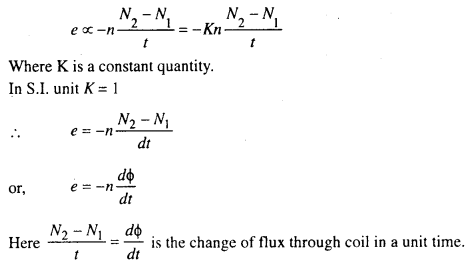Question 9.
What do you understand by alternating current (A.C.)? Obtain an expression for mean value and root mean square (r.m.s.) value of A.C.
Alternating current: A coil rotated in an uniform magnetic field. The induced e.m.f. is produced. In one revolution the value of e.m.f. is zero and maximum in two times. During first half rotation of the coil, current flows in one direction and in next half rotation of direction is reversed. Its magnitude also changes continuously.

Such a current is called “Alternating Current.”
Thus alternating current varies as sine curve. Hence this is called sinusoidal alternating current.
AC. in time t is given by
where i0 is the maximum current and ω is the angular velocity of coil.

Mean value of A.C.: Average value of alternating current in half time period of rotation of coil is called “mean value of A.C.” It is denoted by im.
Time period of A.C. (T) = $$\frac{2 \pi}{\omega}$$
where ω is the angular vel. of coil
∴ Half ime period $$\left(\frac{T}{2}\right)=\frac{\pi}{\omega}$$
Now current produced in time t, i = i0 sin ωt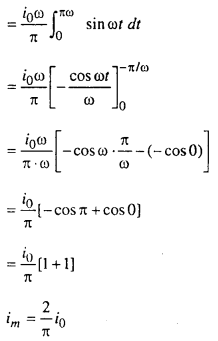i.e. mean value of A.C. = $$\frac{2}{\pi}$$ × max. value of current.

Root Mean square (R.M.S.) value of A.C.: R.M.S. value of A.C. is equal to the square root of the mean of square of current in fully time period of rotation of coil.
It is denoted by irms. It is also called ‘virtual current.’
Now A.C. at time t = i0 sin ωt
∴ square of A.C. at time t = $$i_{0}^{2} \sin ^{2} \omega t d t$$
∴ square of A.C. at time T = $$\int_{0}^{T} i_{0}^{2} \sin ^{2} \omega t d t$$or, ir.m.s = $$\frac{1}{\sqrt{2}} i_{0}$$
or, ir.m.s = 0.707i0
As ir.m.s is also called as virtual current
∴ ivirtual = 0.707i0

Question 10.
Deduce expression for the potential and intensity at a point due to a magnetic dipole.
(a) Potential at a point a magnetic dipole: Let NS be a = (small mangnet or) magnetic dipole of length 2l and magnetic moment M. Its pole strength is m.

Let p be a point distance r from the centre O, of the magnetic dipole in its field where potential is to be calculated.

If the point P is a larger distance from O, then ∠PON and ∠POB will be very small so we can write PA for PN and PB for PS where NA and SB are perpendicular drawn on PO.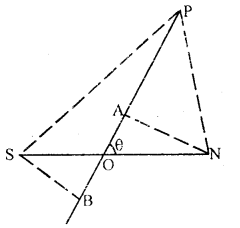Now the potential at P due to N – pole of the dipole.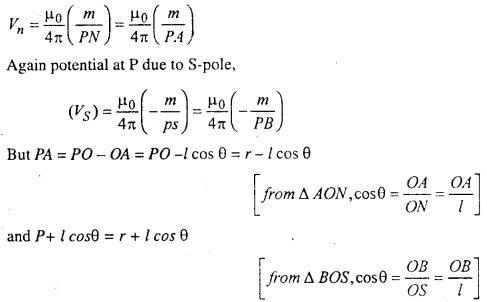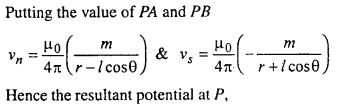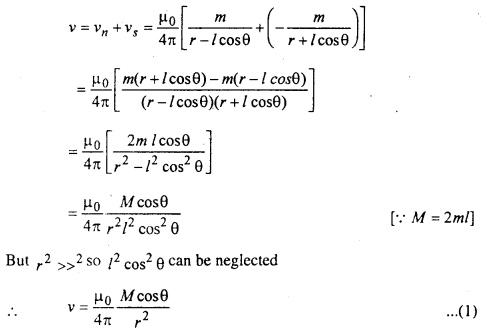This is the required expression for potential at P due to the magnetic dipole.

(b) Magnetic induction at P: We know that potential (v) and magnetic induction (B) are related by the relation B = $$\frac{d v}{d r}$$

Now since v is a function of θ and r, the magnetic induction must have two components, one of which will depend upon the value of θ and the other will depend r the component Bθ, perpendicular to OP will depend on the value of θ.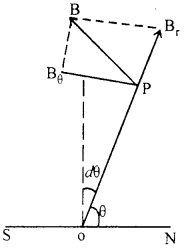Hence the component Br is given by $$\frac{d v}{d r}$$
Since θ is constant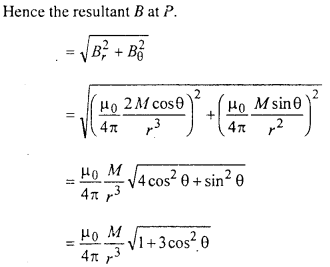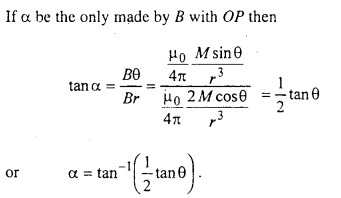Question 11.
State Biot Savart Law. Obtain the magnetic field
(a) At centre of circular loop
(b) At a point due to straight wire.
According to Biot Savart Law the magnetic field dB at a point P associated with an element ‘dl’ has the following properties: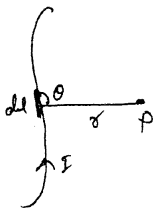1. It is directly proprtional to the current I
∴ dB ∝ I
2. It is directly proportional to the ‘dl’
∴ dB ∝ dl
3. It is directly proportional to sinθ
∴ dB ∝ sinθ
4. It is inversely proportional to the distance of point p.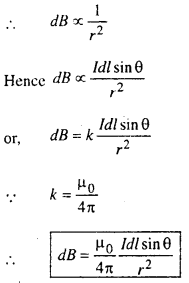(a) Magnetic field at centre of circular loop: A circular loop is shown in the fig. whose centre is o, radius is r and flowing current in It is I.
By Biot Savart Law-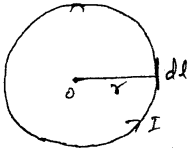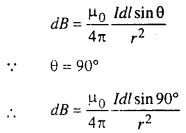(b) Magnetic field at a point due to straight wire:
By Biot Savart Law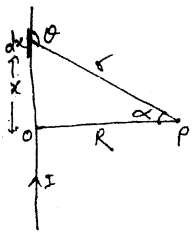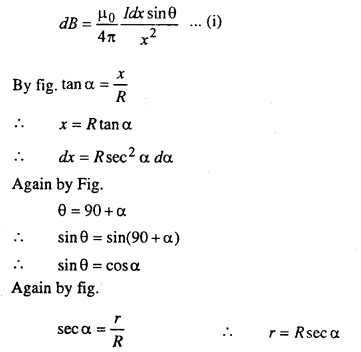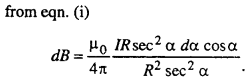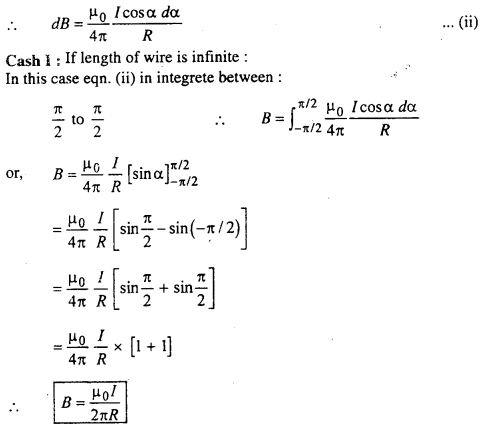Case II: If length or wire is finite:
In this case eqn. (ii) is integrated between – θ2 and θ1.Question 12.
Describe principle and construction of A.C. Dynamo or Generator.
It is a device to convert mechanical energy into electrical energy.
Principle: It is based on the principle of electromagnetic induction. When a coil is rotating in magnetic field then produced e.m.f. and current in coil.

Construction:The construction of A.C. Generator is consists of three parts:
(i) Field Magnet :It is permanent magnet of horse shoe type in a small dynamo where as in large generator. It is an electromagnet. The magnet produces strong magnetic field in which armature rotates.

(ii) Armature: It is a coil of copper wound on an iron drum. It can be rotated, uniformaly about a horizontal axis in the magnetic field of field magnet.

(iii) Slip rings and Carbon brushes: The ends of the coil are connected to two co-axial ring C1 and C2. Carbon brushes K1 and K2 make contact with slip rings. These brushes are connected to external circuit in which current is drawn.

Question 13.
Obtain an expression for refraction of light by spherical surface.
A convex spherical surface is shown in the fig. which is seperated two mediums whose refractive index are μ1 and μ2. Passing rays from object O are formed an image at point I after refraction of surface.
By snell’s law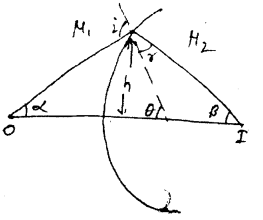$$\frac{\sin i}{\sin r}=\frac{\mu_{2}}{\mu_{1}}$$
∴ $$\frac{i}{r}=\frac{\mu_{2}}{\mu_{1}}$$
∴ iμ1 = rμ2 …………(i)
By fig.
i = α + θ
r = θ – β
from eqn. (i)
(α + θ)μ1 = (θ – β)μ2
or, αμ1 + θμ1 = θμ2 – βμ2
or, βμ2 + aμ1 = θ(μ2 – μ1) ………..(ii)
The Value of α, β and θ are very small so they are represent in form of Tan.Question 14.
Describe the construction and working of a compound microscope with neat diagram obtain an expression for its magnifying power.
Compound microscope is an instrument that magnifies small objects to large one. It consists of two convex lenses placed on the same axis. L1 is of smaller focal length and size, where L2 is of large focal length and size. L1 is placed near the object and is called the objective L2 is near the eye. So it is called eye-piece. Both the lenses are fitted in brass tubes. One tube can slide into the others. The distance of eye-piece can be changed by rack and pinion arrangement. Distance between two lenses is called length of microscope.Let AB be an object to magnify. This is placed at a distance slightly greater than focal of lens. We get a real inverted and larger image A’B’ acts as an object for eye-piece and we get another magnified A”B” we manage eye-piece to get clear image A”B”. In this condition distance of A”B” from eye-piece will be equal to the least distance of distinct vision (D).

Magnifying Power: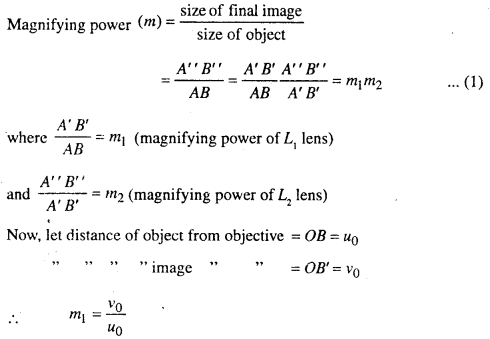For eye piece the distance of object and image will be negative. Hence from lens formula,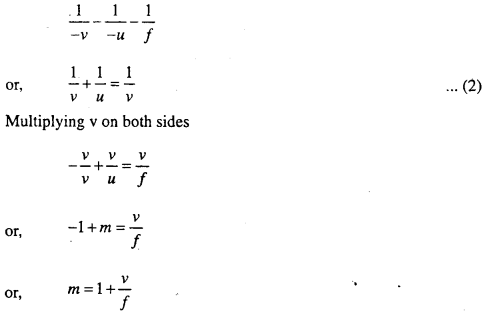Here for eye-piece m = m2 = D and f = F
∴ m2 = 1 + $$\frac{D}{F}$$
Putting the value of m1 and m2 in eqn (1)
m = $$\frac{v_{0}}{u_{0}}\left(1+\frac{D}{F}\right)$$

Magnification will be large if-

1. ν0 is big. It is only possible if length of tubes are large.
2. u0 is small. For this focal length of objective is small.
3. F is small i.e. focal length of eye-piece is small.